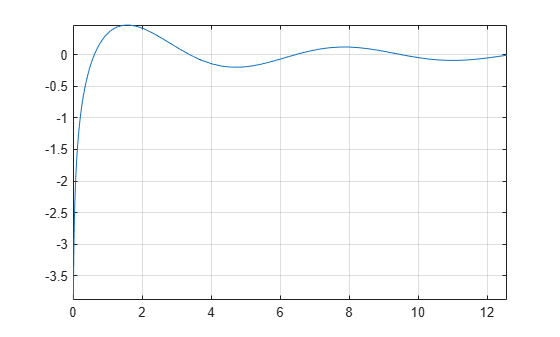# cosint

Cosine integral function

## Syntax

``cosint(X)``

## Description

example

````cosint(X)` returns the cosine integral function of `X`.```

## Examples

### Cosine Integral Function for Numeric and Symbolic Arguments

Depending on its arguments, `cosint` returns floating-point or exact symbolic results.

Compute the cosine integral function for these numbers. Because these numbers are not symbolic objects, `cosint` returns floating-point results.

`A = cosint([- 1, 0, pi/2, pi, 1])`
```A = 0.3374 + 3.1416i -Inf + 0.0000i 0.4720 + 0.0000i... 0.0737 + 0.0000i 0.3374 + 0.0000i```

Compute the cosine integral function for the numbers converted to symbolic objects. For many symbolic (exact) numbers, `cosint` returns unresolved symbolic calls.

`symA = cosint(sym([- 1, 0, pi/2, pi, 1]))`
```symA = [ cosint(1) + pi*1i, -Inf, cosint(pi/2), cosint(pi), cosint(1)]```

Use `vpa` to approximate symbolic results with floating-point numbers:

`vpa(symA)`
```ans = [ 0.33740392290096813466264620388915... + 3.1415926535897932384626433832795i,... -Inf,... 0.47200065143956865077760610761413,... 0.07366791204642548599010096523015,... 0.33740392290096813466264620388915]```

### Plot Cosine Integral Function

Plot the cosine integral function on the interval from 0 to `4*pi`.

```syms x fplot(cosint(x),[0 4*pi]) grid on```### Handle Expressions Containing Cosine Integral Function

Many functions, such as `diff` and `int`, can handle expressions containing `cosint`.

Find the first and second derivatives of the cosine integral function:

```syms x diff(cosint(x), x) diff(cosint(x), x, x)```
```ans = cos(x)/x ans = - cos(x)/x^2 - sin(x)/x```

Find the indefinite integral of the cosine integral function:

`int(cosint(x), x)`
```ans = x*cosint(x) - sin(x)```

## Input Arguments

collapse all

Input, specified as a symbolic number, variable, expression, or function, or as a vector or matrix of symbolic numbers, variables, expressions, or functions.

collapse all

### Cosine Integral Function

The cosine integral function is defined as follows:

`$\text{Ci}\left(x\right)=\gamma +\mathrm{log}\left(x\right)+\underset{0}{\overset{x}{\int }}\frac{\mathrm{cos}\left(t\right)-1}{t}\text{\hspace{0.17em}}dt$`

Here, γ is the Euler-Mascheroni constant:

`$\gamma =\underset{n\to \infty }{\mathrm{lim}}\left(\left(\sum _{k=1}^{n}\frac{1}{k}\right)-\mathrm{ln}\left(n\right)\right)$`

 Gautschi, W. and W. F. Cahill. “Exponential Integral and Related Functions.” Handbook of Mathematical Functions with Formulas, Graphs, and Mathematical Tables. (M. Abramowitz and I. A. Stegun, eds.). New York: Dover, 1972.